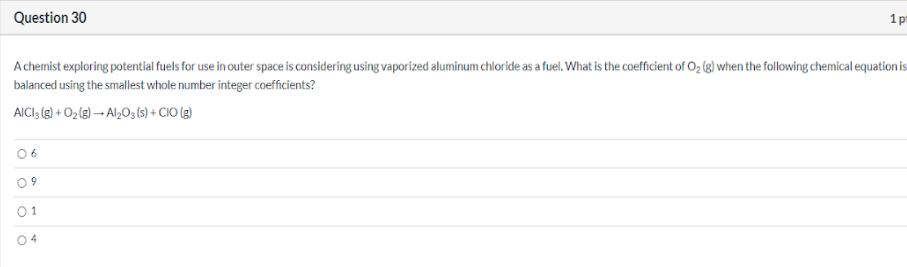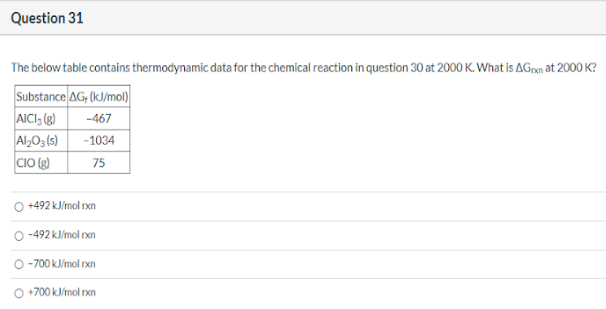# A chemist exploring potential fuels for use in outer space is considering using vaporized aluminum chloride as a fuel. What is the coefficient of O2(g) when the following chemical equation is balanced using the smallest whole number integer coefficients? AlCl3(g) + O2(g) → Al2O3(s) + ClO(g) a) 6 b) 9 c) 1 d) 4 The below table contains thermodynamic data for the chemical reaction in question 30 at 2000 K. What is ΔGrxn at 2000K? a) +492 kJ/mol rxn b) -492 kJ/mol rxn c) -700 kJ/mol rxn d) +700 kJ/mol rxn